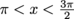# SAT Math: Manipulating the x-Value on a Circle to Find a Positive y-Value

y is a positive real number constant, and x is an angle radian measurement with the restriction. Which of the following changes to x would cause the real number constant to be the opposite sign?

 Additional Topics in Math Congruence and similarity Product Type SAT Math SAT SAT Math SAT Math Additional Topics in MathGeometry and Measurement Test Prep SAT Math